# User:Sankaran/06-1350-HW4

## Contents

### The Generators

Our generators are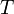$T$,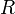$R$,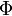$\Phi$ and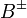$B^{\pm}$:

 Picture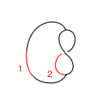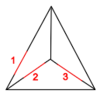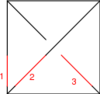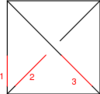Generator$T$$R$$\Phi$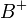$B^+$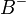$B^-$ Perturbation$t$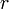$r$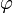$\varphi$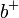$b^+$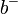$b^-$

(Thanks Zavosh for the nice picture)

### The Relations

#### The Reidemeister Move R2

(Courtesy of Andy)

In formulas, this is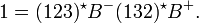$1 = (123)^\star B^- (132)^\star B^+.$

Linearized and written in functional form, this becomes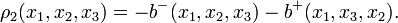$\rho_2(x_1,x_2,x_3) = - b^-(x_1,x_2,x_3) - b^+(x_1,x_3,x_2).$

#### The Reidemeister Move R3

(Picture and first example courtesy of Dror)

There are eight of these (each crossing in the picture can be + or - ). For example, if all the crossings are positive, the picture (with three sides of the shielding removed) is

In formulas, this is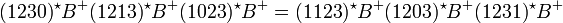$(1230)^\star B^+ (1213)^\star B^+ (1023)^\star B^+ = (1123)^\star B^+ (1203)^\star B^+ (1231)^\star B^+$.

Linearized and written in functional form, this becomes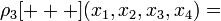$\rho_3[+++](x_1, x_2, x_3, x_4) =$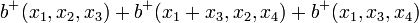$b^+(x_1,x_2,x_3) + b^+(x_1+x_3,x_2,x_4) + b^+(x_1,x_3,x_4)$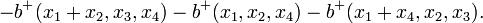$- b^+(x_1+x_2,x_3,x_4) - b^+(x_1,x_2,x_4) - b^+(x_1+x_4,x_2,x_3).$

Here are the rest of them, linearized and in functional form - I think this is too many, but it's probably easier to write these out than to figure the relationships between them. Also, some better notation is needed.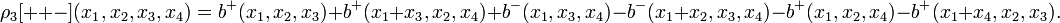$\rho_3[++-](x_1,x_2,x_3,x_4) = b^+(x_1,x_2,x_3) + b^+(x_1+x_3,x_2,x_4) + b^-(x_1,x_3,x_4) - b^-(x_1+x_2,x_3,x_4) - b^+(x_1,x_2,x_4) - b^+(x_1+x_4,x_2,x_3).$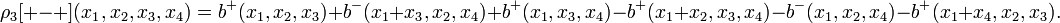$\rho_3[+-+](x_1,x_2,x_3,x_4) = b^+(x_1,x_2,x_3) + b^-(x_1+x_3,x_2,x_4) + b^+(x_1,x_3,x_4)- b^+(x_1+x_2,x_3,x_4) - b^-(x_1,x_2,x_4) - b^+(x_1+x_4,x_2,x_3).$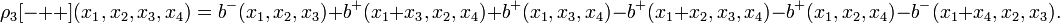$\rho_3[-++](x_1,x_2,x_3,x_4) = b^-(x_1,x_2,x_3) + b^+(x_1+x_3,x_2,x_4) + b^+(x_1,x_3,x_4)- b^+(x_1+x_2,x_3,x_4) - b^+(x_1,x_2,x_4) - b^-(x_1+x_4,x_2,x_3).$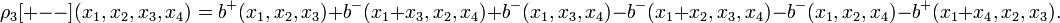$\rho_3[+--](x_1,x_2,x_3,x_4) = b^+(x_1,x_2,x_3) + b^-(x_1+x_3,x_2,x_4) + b^-(x_1,x_3,x_4)- b^-(x_1+x_2,x_3,x_4) - b^-(x_1,x_2,x_4) - b^+(x_1+x_4,x_2,x_3).$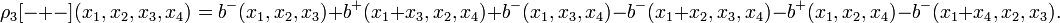$\rho_3[-+-](x_1,x_2,x_3,x_4) = b^-(x_1,x_2,x_3) + b^+(x_1+x_3,x_2,x_4) + b^-(x_1,x_3,x_4)- b^-(x_1+x_2,x_3,x_4) - b^+(x_1,x_2,x_4) - b^-(x_1+x_4,x_2,x_3).$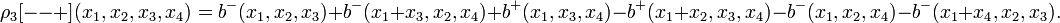$\rho_3[--+](x_1,x_2,x_3,x_4) = b^-(x_1,x_2,x_3) + b^-(x_1+x_3,x_2,x_4) + b^+(x_1,x_3,x_4)- b^+(x_1+x_2,x_3,x_4) - b^-(x_1,x_2,x_4) - b^-(x_1+x_4,x_2,x_3).$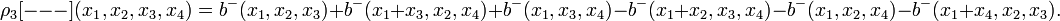$\rho_3[---](x_1,x_2,x_3,x_4) = b^-(x_1,x_2,x_3) + b^-(x_1+x_3,x_2,x_4) + b^-(x_1,x_3,x_4)- b^-(x_1+x_2,x_3,x_4) - b^-(x_1,x_2,x_4) - b^-(x_1+x_4,x_2,x_3).$

#### The Reidemeister Move R4

(Courtesy of Andy)

There are two (ostensibly) different versions:

In formulas, this is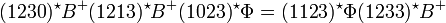$(1230)^\star B^+ (1213)^\star B^+ (1023)^\star \Phi = (1123)^\star \Phi (1233)^\star B^+$.

Linearized and written in functional form, this becomes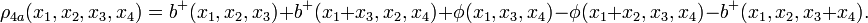$\rho_{4a}(x_1,x_2,x_3,x_4) = b^+(x_1,x_2,x_3) + b^+(x_1+x_3,x_2,x_4) + \phi(x_1,x_3,x_4) - \phi(x_1+x_2,x_3,x_4) - b^+(x_1,x_2,x_3+x_4).$

Second:

In formulas, this is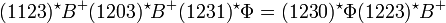$(1123)^\star B^+ (1203)^\star B^+ (1231)^\star \Phi = (1230)^\star \Phi (1223)^\star B^+$.

Linearized and written in functional form, this becomes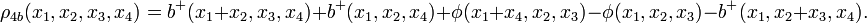$\rho_{4b}(x_1,x_2,x_3,x_4) = b^+(x_1+x_2,x_3,x_4) + b^+(x_1,x_2,x_4) + \phi(x_1+x_4,x_2,x_3) - \phi(x_1,x_2,x_3) - b^+(x_1,x_2+x_3,x_4).$

### The Syzygies

#### The "B around B" Syzygy

The picture, with all shielding removed, is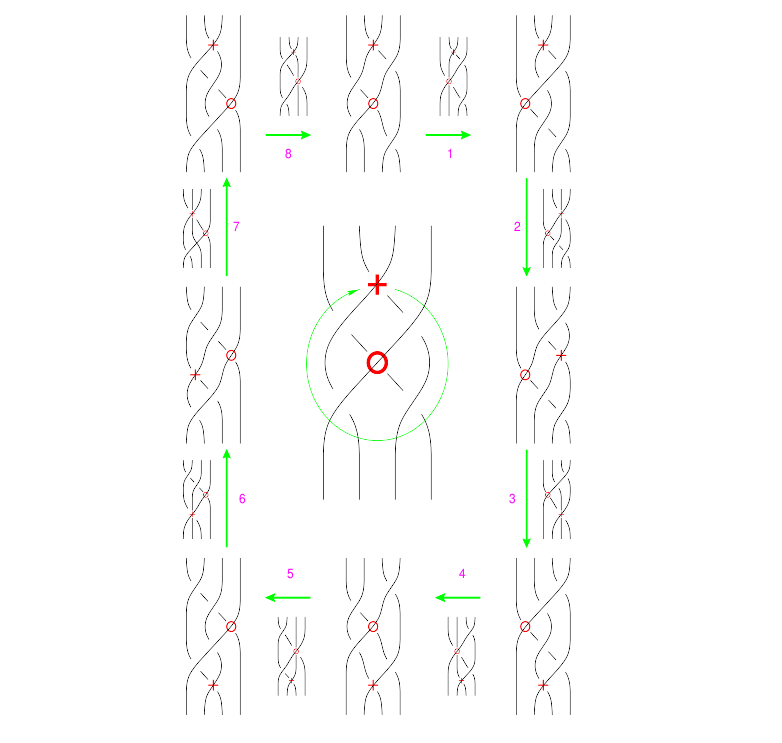(Drawn with Inkscape)(note that lower quality pictures are also acceptable)

The functional form of this syzygy is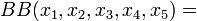$BB(x_1,x_2,x_3,x_4,x_5) =$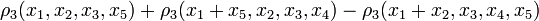$\rho_3(x_1, x_2, x_3, x_5) + \rho_3(x_1 + x_5, x_2, x_3, x_4) - \rho_3(x_1 + x_2, x_3, x_4, x_5)$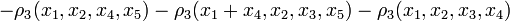$- \rho_3(x_1, x_2, x_4, x_5) - \rho_3(x_1 + x_4, x_2, x_3, x_5) - \rho_3(x_1, x_2, x_3, x_4)$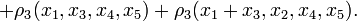$+ \rho_3(x_1, x_3, x_4, x_5) + \rho_3(x_1 + x_3, x_2, x_4, x_5).$

### A Mathematica Verification

The following simulated Mathematica session proves that for our single relation and single syzygy,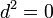$d^2=0$. Copy paste it into a live Mathematica session to see that it's right!

 In:= d1 = { rho3[x1_, x2_, x3_, x4_] :> bp[x1, x2, x3] + bp[x1 + x3, x2, x4] + bp[x1, x3, x4] - bp[x1 + x2, x3, x4] - bp[x1, x2, x4] - bp[x1 + x4, x2, x3] }; d2 = { BAroundB[x1_, x2_, x3_, x4_, x5_] :> rho3[x1, x2, x3, x5] + rho3[x1 + x5, x2, x3, x4] - rho3[x1 + x2, x3, x4, x5] - rho3[x1, x2, x4, x5] - rho3[x1 + x4, x2, x3, x5] - rho3[x1, x2, x3, x4] + rho3[x1, x3, x4, x5] + rho3[x1 + x3, x2, x4, x5] };
 In:= BAroundB[x1, x2, x3, x4, x5] /. d2 Out= - rho3[x1, x2, x3, x4] + rho3[x1, x2, x3, x5] - rho3[x1, x2, x4, x5] + rho3[x1, x3, x4, x5] - rho3[x1 + x2, x3, x4, x5] + rho3[x1 + x3, x2, x4, x5] - rho3[x1 + x4, x2, x3, x5] + rho3[x1 + x5, x2, x3, x4]
 In:= BAroundB[x1, x2, x3, x4, x5] /. d2 /. d1 Out= 0